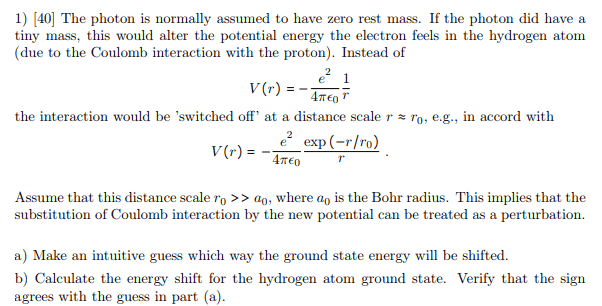# Perturbation for Yukawa Potential

Warda Anis

## Homework Statement

The photon is normally assumed to have zero rest mass. If the photon did have a tiny mass, this would alter the potential energy the electron feels in the hydrogen atom (due to the Coulomb interaction with the proton). The potential then becomes yukawa potential.V(r)=-e^2/4pi*epsilon (exp(-r/r0))/r
Assume that this distance scale r0 >> a0, where a0 is the Bohr radius. This implies that the substitution of Coulomb interaction by the new potential can be treated as a perturbation.
a) Make an intuitive guess which way the ground state energy will be shifted.
b) Calculate the energy shift for the hydrogen atom ground state. Verify that the sign agrees with the guess in part (a).

## The Attempt at a Solution

So I know that energy shift is,
ΔEo=λ<Ψo|H'|Ψo>
where,
Ψo=sqrt(Z^3/π)exp(-Zr)
H'= − e^2/(4πε) exp (−r/ro)
Could anyone please help me with the second part of the question? How should I proceed from here?

#### Attachments

Last edited:

Welcome to the PF.EDIT -- I see you gone back and added more information in your OP. Thank you.